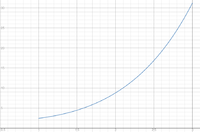# Particle Motion along y-axis at time t > 0 seconds is given by the function y(t)=2e^t-3t

#### Browns21284

##### New member
The position of a particle moving along the y-axis at time t > 0 seconds is given by the function y(t)=2e^t-3t
a. Find the average velocity of the particle over the interval [1, 3]. Be sure to indicate correct units of measure.
b. In what direction is the particle moving and what is the particle’s velocity at t = 2 seconds? Justify your answers and be sure to indicate correct units of measure.
c. What is the acceleration of the particle when the velocity is zero? Show the analysis that leads to your answer and be sure to indicate correct units of measure.
d. Is the speed increasing or decreasing at t = 1.5 seconds? Justify your answer.

Thanks a lot for the help!

#### MarkFL

##### Super Moderator
Staff member
Hello, and welcome to FMH!Can you show what you've done so far, so we know where you're stuck?

•topsquark

#### HallsofIvy

##### Elite Member
First, do you know the definitions of the words you are using? What does "average speed" over a given time interval mean? What does the "speed at a given instant" mean?

#### Subhotosh Khan

##### Super Moderator
Staff member
The position of a particle moving along the y-axis at time t > 0 seconds is given by the function y(t)=2e^t-3t
a......... Be sure to indicate correct units of measure.
b. ............................ indicate correct units of measure.
c. ............................ indicate correct units of measure.
d. Is the speed increasing or decreasing at t = 1.5 seconds? Justify your answer.

Thanks a lot for the help!
What is the unit of measure of 'y'?

•topsquark

#### MarkFL

##### Super Moderator
Staff member
Let's first look at a graph of the particle's position over the indicated interval:Now, we are told the particle's position on the $$y$$-axis is given by:

$$\displaystyle y(t)=2e^t-3t$$

And thus, the velocity is given by:

$$\displaystyle v(t)=\d{y}{t}$$

Hence, the average velocity over the interval is:

$$\displaystyle \overline{v(t)}=\frac{1}{3-1}\int_1^3 v(t)\,dt=\frac{1}{2}\int_1^3\,dy=\frac{y(3)-y(1)}{2}=?$$

Once you get that, we can move on to part b).